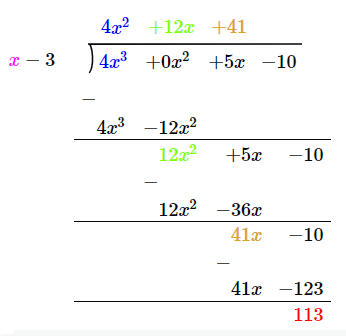QUESTION

# Using the remainder theorem, find the remainder when $4{{x}^{3}}+5x-10$ is divisible by $\left( x-3 \right)$.A. 12B. 18C. 96D. 113

Hint: Use the remainder theorem. We have been given f(x) and d(x). Thus using a long division method for polynomials, find the quotient and remainder of the given f(x).

The remainder theorem states that when a polynomial f(x) is divided by a linear polynomial $\left( x-a \right)$ then the remainder of that division will be equal to f(a).
If you want to evaluate the function f(x) for a given number a, you can divide the function by $\left( x-a \right)$ and your remainder will be equal to f(a).
Now we have been given $f(x)=4{{x}^{3}}+5x-10$ to be divided by $\left( x-3 \right)$ by long division method.
For e.g.:- if we are dividing 7 by 2, we get remainder 1.
$7\div 2=3$, R = 1.
7 divided by 2 equals 3 with remainder 1, where 7 is dividend, 2 is divisor, 3 is quotient and 1 is remainder.
$\therefore 7=2\times 3+1$.
$\therefore f(x)\div d(x)=q(x)$ with a remainder r(x).
$f(x)=d(x).q(x)+r(x)$
Thus the polynomials can be written in this form.
$\therefore f(x)=4{{x}^{3}}+5x-10$ and $d(x)=x-3$First find $4{{x}^{2}}(x-3)=4{{x}^{3}}-12{{x}^{2}}$ as to get the first term. Then we get the remainder as $\left( 12{{x}^{2}}+5x \right)$. Now multiply $12x\left( x-3 \right)$ to get $12{{x}^{2}}-36x.$ We get the remainder as $\left( 41x-10 \right).$
Now do $41(x-3)=41x-123.$
Thus we get the final remainder as 113.
\begin{align} & f(x)=4{{x}^{3}}+5x-10 \\ & d(x)=(x-3) \\ & q(x)=4{{x}^{2}}+12x+41 \\ & r(x)=113. \\ \end{align}
Thus we got the remainder as 113, using the remainder theorem.
Option D is the correct answer.

Note:
We should know that the remainder theorem only works when a function is divided by a linear polynomial. It should be of the form (x + number) or (x - number). For e.g.:- (x + a) or (x - a), where a can be any integer.